Study Materials: ncert solutions

Our ncert solutions for Exercise 1.2 Class 10 maths 1. Real Numbers - ncert solutions - Toppers Study is the best material for English Medium students cbse board and other state boards students.

Solutions ⇒ Class 10th ⇒ Mathematics ⇒ 1. Real Numbers

# Exercise 1.2 Class 10 maths 1. Real Numbers - ncert solutions - Toppers Study

Topper Study classes prepares ncert solutions on practical base problems and comes out with the best result that helps the students and teachers as well as tutors and so many ecademic coaching classes that they need in practical life. Our ncert solutions for Exercise 1.2 Class 10 maths 1. Real Numbers - ncert solutions - Toppers Study is the best material for English Medium students cbse board and other state boards students.

## Exercise 1.2 Class 10 maths 1. Real Numbers - ncert solutions - Toppers Study

CBSE board students who preparing for class 10 ncert solutions maths and Mathematics solved exercise chapter 1. Real Numbers available and this helps in upcoming exams 2023-2024.

### You can Find Mathematics solution Class 10 Chapter 1. Real Numbers

• All Chapter review quick revision notes for chapter 1. Real Numbers Class 10
• NCERT Solutions And Textual questions Answers Class 10 Mathematics
• Extra NCERT Book questions Answers Class 10 Mathematics
• Importatnt key points with additional Assignment and questions bank solved.

NCERT Solutions do not only help you to cover your syllabus but also will give to textual support in exams 2023-2024 to complete exercise 1.2 maths class 10 chapter 1 in english medium. So revise and practice these all cbse study materials like class 10 maths chapter 1.2 in english ncert book. Also ensure to repractice all syllabus within time or before board exams for ncert class 10 maths ex 1.2 in english.

See all solutions for class 10 maths chapter 1 exercise 1 in english medium solved questions with answers.

### Exercise 1.2 class 10 Mathematics Chapter 1. Real Numbers

• Exercise 1.2 Class 10 Maths 1. Real Numbers - Ncert Solutions - Toppers Study
• Class 10 Ncert Solutions
• Solution Chapter 1. Real Numbers Class 10
• Solutions Class 10
• Chapter 1. Real Numbers Exercise 1.2 Class 10

## Exercise 1.2 Class 10 maths 1. Real Numbers - ncert solutions - Toppers Study

EXERCISE 1.2 Chapter 1. Real Numbers class 10 Maths

Q1. Express each number as a product of its prime factors:
(i) 140

Solution: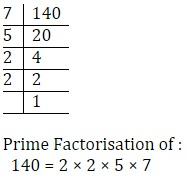= 22 × 5 × 7

(ii) 156

Solution: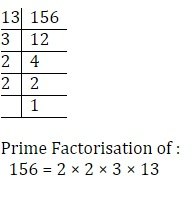= 22 × 3 × 13

(iii) 3825

Solution: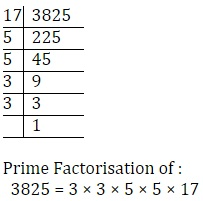= 32 × 52 × 17

(iv) 5005

Solution: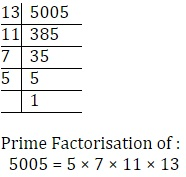= 5 × 7 × 11 × 13

(v) 7429

Solution: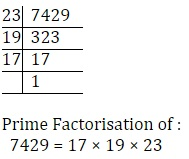Q2. Find the LCM and HCF of the following pairs of integers and verify that LCM × HCF = product of the two numbers.

(i) 26 and 91

Solution:

26 = 2 × 13

91 = 7 × 13

Common factors = 13

∴ HCF = 13

LCM = 2 × 7 × 13 = 182

Now varification,

product of the two numbers = LCM × HCF

N1 × N2LCM × HCF

26 × 91 = 13 × 182

2366 =  2366

Hence Varified,

(ii) 510 and 92

Solution:

510 = 2 × 3 × 5 × 17

92 = 2 × 2 × 23

Common factors = 2

∴ HCF = 2

LCM = 2 × 2 × 3 × 5 × 17 × 23 =  23460

Now varification,

product of the two numbers = LCM × HCF

N1 × N2 = LCM × HCF

510 × 92 = 2 × 23460

46920 =  46920

Hence Varified,

(iii) 336 and 54

Solution:

336 = 2 × 2 × 2 × 2 × 3 × 7

54 = 2 × 3 × 3 × 3

Common factors = 2 × 3

∴ HCF = 6

LCM = 2 × 2 × 2× 2 × 3 × 3 × 3 × 7 =  3024

Now varification,

product of the two numbers = LCM × HCF

N1 × N2 = LCM × HCF

336 × 54 = 6 × 3024

18144 =  18144

Hence Varified,

Q3. Find the LCM and HCF of the following integers by applying the prime factorisation method.

(i) 12, 15 and 21

Solution:

12 = 2 × 2 × 3

15 = 5 × 3

21 = 7 × 3

Common Factors = 3

HCF = 3

​LCM = 3 × 2 × 2 × 5 × 7 = 420

(ii) 17, 23 and 29

Solution:

17 = 1 × 17

23 = 1 × 23

29 = 1 × 29

HCF = 1

LCM = 17 × 23 × 29 = 11339

(iii) 8, 9 and 25

Solution:

8 = 2 × 2 × 2

9 = 3 × 3

25 = 5 × 5

There is no common factor except 1.

∴ HCF = 1

LCM = 2 × 2 × 2 × 3 × 3 × × 5

= 8 × 9 × 25

= 1800

Q4. Given that HCF (306, 657) = 9, find LCM (306, 657).

Solution:

HCF (306, 657) = 9

LCM × HCF = ​N1 × N2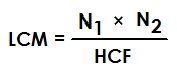LCM = 22338

Q5. Check whether 6n can end with the digit 0 for any natural number n.

Solution:

Prime factorisation of 6n = (2 × 3 )n

While, Any natural number which end with digit 0 has

the prime factorisation as form of (2 × 5 )n

Therefore, 6n will not end with digit 0.

Q6. Explain why 7 × 11 × 13 + 13 and 7 × 6 × 5 × 4 × 3 × 2 × 1 + 5 are composite numbers.

Solution:

Let A = 7 × 11 × 13 + 13

= 13 (7 × 11 + 1)

= 13 (77 + 1)

= 13 × 78

Hence this is composite number because It has at least one positive divisor other than one.

Similarily,

Let B = 7 × 6 × 5 × 4 × 3 × 2 × 1 + 5

= 5 (7 × 6 × 4 × 3 × 2 × 1 + 1)​

= 5 × (1008 + 1)

= 5  ×  1009

Hence this is also a composite number because It has at least one positive divisor other than one.

Q7. There is a circular path around a sports field. Sonia takes 18 minutes to drive one round of the field, while Ravi takes 12 minutes for the same. Suppose they both start at the same point and at the same time, and go in the same direction. After how many minutes will they meet again at the starting point?

Solution:

Sonia takes 18 minutes in one round.

Ravi takes 12 minutes in one round

they will meet again at the starting point after LCM(18, 12) minutes

18 = 2 × 3 × 3

12 = 2 × 2 × 3

HCF = 2 × 3 = 6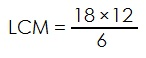= 36 minutes

##### Other Pages of this Chapter: 1. Real Numbers

Important Study materials for classes 06, 07, 08,09,10, 11 and 12. Like CBSE Notes, Notes for Science, Notes for maths, Notes for Social Science, Notes for Accountancy, Notes for Economics, Notes for political Science, Noes for History, Notes For Bussiness Study, Physical Educations, Sample Papers, Test Papers, Mock Test Papers, Support Materials and Books.Mathematics Class - 11th

NCERT Maths book for CBSE Students.

books

## Study Materials List:

##### Solutions ⇒ Class 10th ⇒ Mathematics
1. Real Numbers
2. Polynomials
3. Pair of Linear Equations in Two Variables
5. Arithmetic Progressions
6. Triangles
7. Coordinate Geometry
8. Introduction to Trigonometry
9. Some Applications of Trigonometry
10. Circles
11. Constructions
12. Areas Related to Circles
13. Surface Areas and Volumes
14. Statistics
15. Probability

New Books